# Normal sheaf

An analogue to a normal bundle in sheaf theory. Let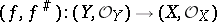be a morphism of ringed spaces such that the homomorphism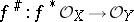is surjective, and let. Thenis a sheaf of ideals inand is, therefore, an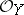-module. Here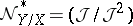is called the conormal sheaf of the morphism and the dual-module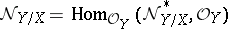is called the normal sheaf of the morphism. These sheaves are, as a rule, examined in the following special cases.

1)andare differentiable manifolds (for example, of class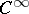), andis an immersion. There is an exact sequence of-modules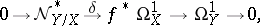where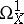andare the sheaves of germs of smooth-forms onand, andis defined as differentiation of functions. The dual exact sequencewhere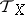and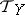are the tangent sheaves onand, shows that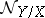is isomorphic to the sheaf of germs of smooth sections of the normal bundle of the immersion. Ifis an immersed submanifold, thenand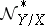are called the normal and conormal sheaves of the submanifold.

2)is an irreducible separable scheme of finite type over an algebraically closed field,is a closed subscheme of it andis an imbedding. Thenandare called the normal and conormal sheaves of the subscheme. There is also an exact sequence of-modules(*)

whereand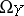are the sheaves of differentials onand. The sheavesandare quasi-coherent, and ifis a Noetherian scheme, then they are coherent. Ifis a non-singular variety overandis a non-singular variety, thenis locally free and the homomorphismin (*) is injective. In this case one obtains the dual exact sequence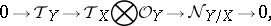so that the normal sheafis locally free of rank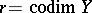corresponding to the normal bundle over. In particular, if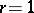, thenis the invertible sheaf corresponding to the divisor.

In terms of normal sheaves one can express the self-intersectionof a non-singular subvariety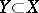. Namely,, where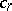is the-th Chern class and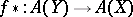is the homomorphism of Chow rings (cf. Chow ring) corresponding to the imbedding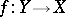.

3)is a complex space,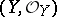is a closed analytic subspace of it andis the imbedding. Thenandare called the normal and conormal sheaves of the subspace; they are coherent. Ifis an analytic manifold andan analytic submanifold of it, thenis the sheaf of germs of holomorphic sections of the normal bundle over.

How to Cite This Entry:
Normal sheaf. Encyclopedia of Mathematics. URL: http://encyclopediaofmath.org/index.php?title=Normal_sheaf&oldid=23917
This article was adapted from an original article by A.L. Onishchik (originator), which appeared in Encyclopedia of Mathematics - ISBN 1402006098. See original article